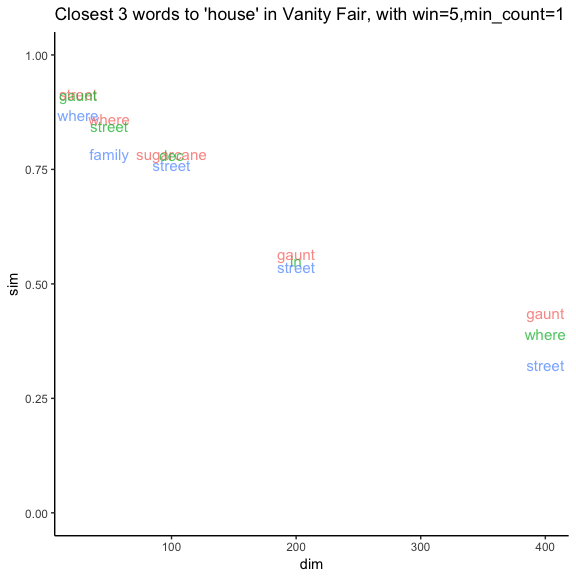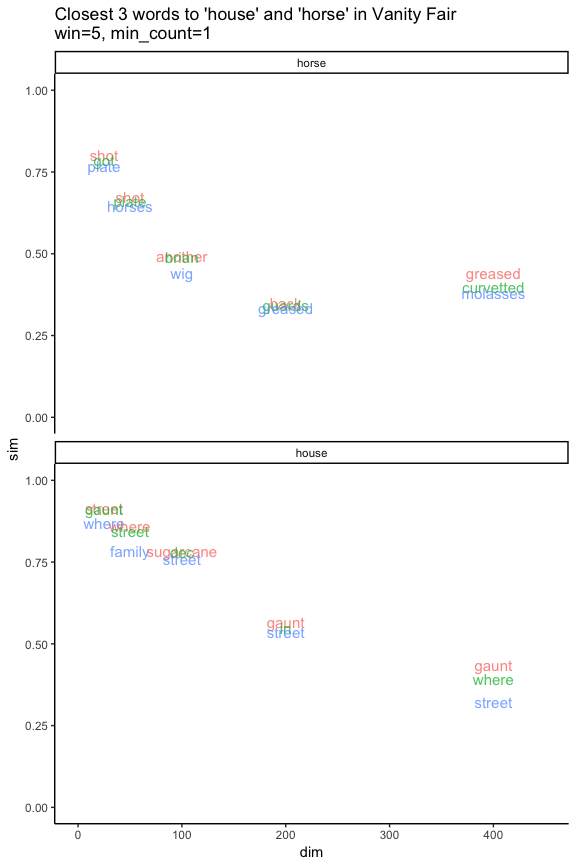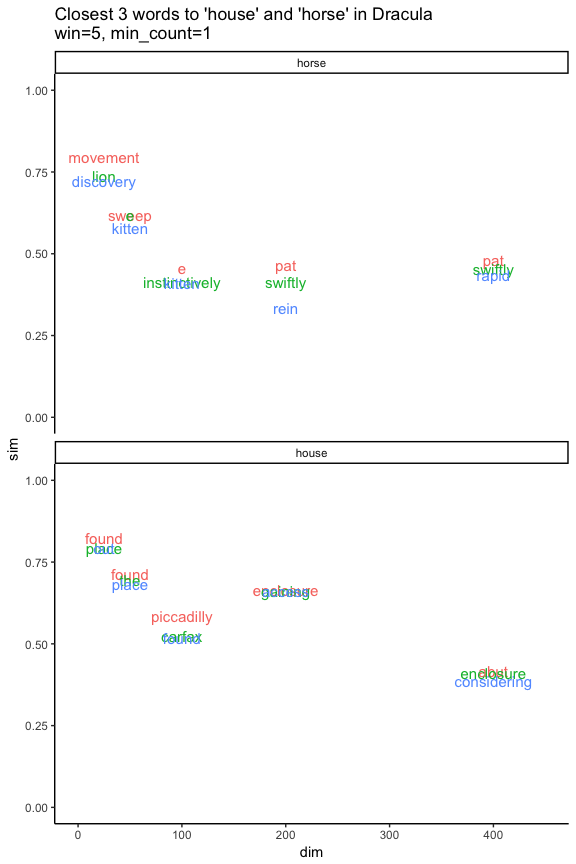Overview

This is one of a series of posts on using word vectors with small corpora. In this post I discuss ways to explore word similarities that don’t compare the model results with precompiled human judgments. In particular, I show how we can use “the closests of the closests” as well as the slope of similar items to get different perspectives on word similarities, both within a corpus and across corpora.

library(tidyverse)
library(scales)
library(reticulate)
prep = FALSE
# set up python functions
if (prep) {
use_condaenv("textp") #this must have gensim installed

psys <- import('sys')
psys\$path <- c(psys\$path,getwd())

svd_similarity <- import("similarities")

get_most_similar <- function(text,words,n,win,dim,min_count) {
x <- svd_similarity\$get_most_similar(text,words,n=n,win=win,dim=dim,min_count=min_count)

nr = length(x)
what <- data.frame(matrix(unlist(x), nrow=nr, byrow=T),stringsAsFactors=FALSE)
names(what) <- c('text','word','win','dim','min_count','item','rank','sim')

return(what)
}
}
# calculate/load  data that needs python
if (prep) {
text <- "vfair"
n <- 10
min_count <- NULL

vfair_sims <-
get_most_similar(text,words,n=n,win=NULL,dim=NULL,min_count=min_count)
write_tsv(vfair_sims,"sims/vfair_sims.csv")
}
if (prep) {
text <- "waywe"
n <- 10
min_count <- 1
words <- c("house","horse")
waywe_house_horse <-
get_most_similar(text,words,n=n,win=NULL,dim=NULL,min_count=min_count)
write_tsv(waywe_house_horse,"sims/waywe_house_horse.csv")
}
if (prep) {
text <- "moby"
n <- 10
min_count <- 1
words <- c("house","horse")
moby_house_horse <-
get_most_similar(text,words,n=n,win=NULL,dim=NULL,min_count=min_count)
write_tsv(moby_house_horse,"sims/moby_house_horse.csv")
}
if (prep) {
text <- "kidnapped"
n <- 10
min_count <- 1
words <- c("house","horse")
kidnapped_house_horse <-
get_most_similar(text,words,n=n,win=NULL,dim=NULL,min_count=min_count)
write_tsv(kidnapped_house_horse,"sims/kidnapped_house_horse.csv")
}
if (prep) {
text <- "dracula"
n <- 10
words <- c("house","horse","life","awful")
dracula_sims <-
get_most_similar(text,words,n=n,win=NULL,dim=NULL,min_count=NULL)
write_tsv(dracula_sims,"sims/dracula_sims.csv")
}
if (prep) {
text <- "jane"
words <- c("house","horse","life","awful")
jane_sims <- get_most_similar(text,words,n=n,win=NULL,dim=NULL,min_count=NULL)
write_tsv(jane_sims, "sims/jane_sims.csv")
}
if (prep) {
text <- "threemen"
words <- c("house","horse","life","awful")
threemen_sims <- get_most_similar(text,words,n=n,win=NULL,dim=NULL,min_count=NULL)
write_tsv(threemen_sims, "sims/threemen_sims.csv")
}
###############
cnames <- list('davidc', 'rbadge', 'dracula', 'moby', 'scarlet', 'emma', 'moonstone', 'frankenstein', 'pym', 'sybil', 'heartd', 'grubb', 'threemen', 'jane', 'nabbey', 'vfair', 'dorian', 'waywe', 'kidnapped', 'wuthering')
eval_texts <- list('sign4','jude','midmarch')
test_names <- list("ws353","ws353_similarity","ws353_relatedness","bruni_men")
counts <- Reduce(rbind,lapply(cnames, function(c){
fname <- paste0("counts/",c,"-counts.csv")
these_counts <- read_delim(fname, "\t", escape_double = FALSE, trim_ws = TRUE) %>%
mutate(text=c, rank=row_number())

#now add rank percentile [NOT count percentile, which isn't useful]
len <- nrow(these_counts)
these_counts %>% mutate(percentile=round(100*(1-rank/len), digits=2))

})) %>% select(text, everything())
best_SVD_sim_scores <- read_delim("testsets_parameters/SVD-sim_tests.csv", "\t", escape_double = FALSE, trim_ws = TRUE) %>%
filter(!(text %in% eval_texts)) %>%
group_by(testset,text) %>%
filter(sF1 == max(sF1))

Background

In the previous post, Finding useful parameters and methods, I discussed finding the values for the parameters window size (win), number of dimensions (dim), and the minimum frequency of words to be included (min_count) that give the highest sF1 scores on four standard testsets of human similarity judgments. However, as mentioned at the end of that post, it is reasonable to question whether those testsets are relevant to evaluating these small corpora (recall that I am using 19th century novels as my corpora).

One problem with the testsets in this context is that they contain a lot of vocabulary that is not found in any single text. We saw that the best recall was still less than 75%, and with smaller texts it was not uncommon for recall to be less than 30%. The testsets then tell us little about the vocabulary that is in the texts.

A second problem with the testsets is that they reflect 21st century judgments about word usage, and we know that word usage (and meaning) has changed since the 19th century. In fact, there have been recent papers using word vectors to quantify those changes (, , ). In other words, the testsets can only tell us about 21st century interpretations of 19th century usage. While that might be interesting in its own right, it is not a way to discover how 19th century authors used words.

A third issue in using word vectors to explore word similarities is that different parameter settings give different similarities. Here is one example from Vanity Fair. I’ve chosen the word house, as the most common noun (lady is more common but it can also be a title, as in lady jane, since we have lowercased everything in the preprocessing.) I’ve fixed the window size at 5, and for each dimension in the parameters experiment, we see the 3 words that the model judges as being the most similar to house.

Throughout this post, as in the parameters experiment, I am using ppmi_svd vectors created using hyperwords , with similarities calculated using gensim ).

vfair_house_horse <- vfair_sims %>% filter(min_count==1,word=='house' | word=='horse')
vfair_house_horse %>%
filter(word=='house',win==5, rank<4) %>%
ggplot() + theme_classic() +
labs(title="Closest 3 words to 'house' in Vanity Fair, with win=5,min_count=1") +
scale_y_continuous(limits = c(0,1)) +
geom_text(aes(dim,sim,label=item, color=factor(rank)), alpha=0.75, size=4, show.legend = FALSE)We can immediately see two issues:

• the most similar words vary from setting to setting
• the similarity (sim) varies from setting to setting

A third issue is that although win=4, dim=400 was the best scoring setting for the testsets, it gives the lowest similarity scores for house. In other words, a model that does well on the testsets will not necessarily give the highest similarity scores.

If we add in the closest words to horse, we see that similarity scores across words varies, even within the same settings. For example, the closest words to horse with dim=25 have a similarity of a little more than 0.75, while for house the scores are closer to 0.9.

vfair_house_horse %>%
filter(win==5, rank<4) %>%
ggplot() + theme_classic() +
labs(title="Closest 3 words to 'house' and 'horse' in Vanity Fair\nwin=5, min_count=1") +
scale_y_continuous(limits = c(0,1)) +
scale_x_continuous(limits = c(0,450)) +
geom_text(aes(dim,sim,label=item, color=factor(rank)), size=4, alpha=0.75, show.legend = FALSE) +
facet_wrap(~word, ncol=1)Finally, when we look at a different text, Dracula, we see different words (not surprisingly), and different scores. There is also a slight difference in the trends of the scores across the dimensions.

dracula_house_horse <- dracula_sims %>% filter(min_count==1,word=='house' | word=='horse')
dracula_house_horse %>%
filter(win==5, rank<4) %>%
ggplot() + theme_classic() +
labs(title="Closest 3 words to 'house' and 'horse' in Dracula\nwin=5, min_count=1") +
scale_y_continuous(limits = c(0,1)) +
scale_x_continuous(limits = c(0,450)) +
geom_text(aes(dim,sim,label=item, color=factor(rank)), size=4, show.legend = FALSE) +
facet_wrap(~word, ncol=1)rbind(vfair_house_horse,dracula_house_horse) %>%
filter(win==5, rank==1) %>%
ggplot() + theme_classic() +
labs(title="Trends for closest word to house, horse\nin Vanity Fair, Dracula, win=5, min_count=1") +
scale_y_continuous(limits = c(0,1)) +
geom_line(aes(dim,sim,color=text)) +
geom_point(aes(dim,sim,color=text, shape=text), size=2) +
facet_wrap(~word, ncol=1)